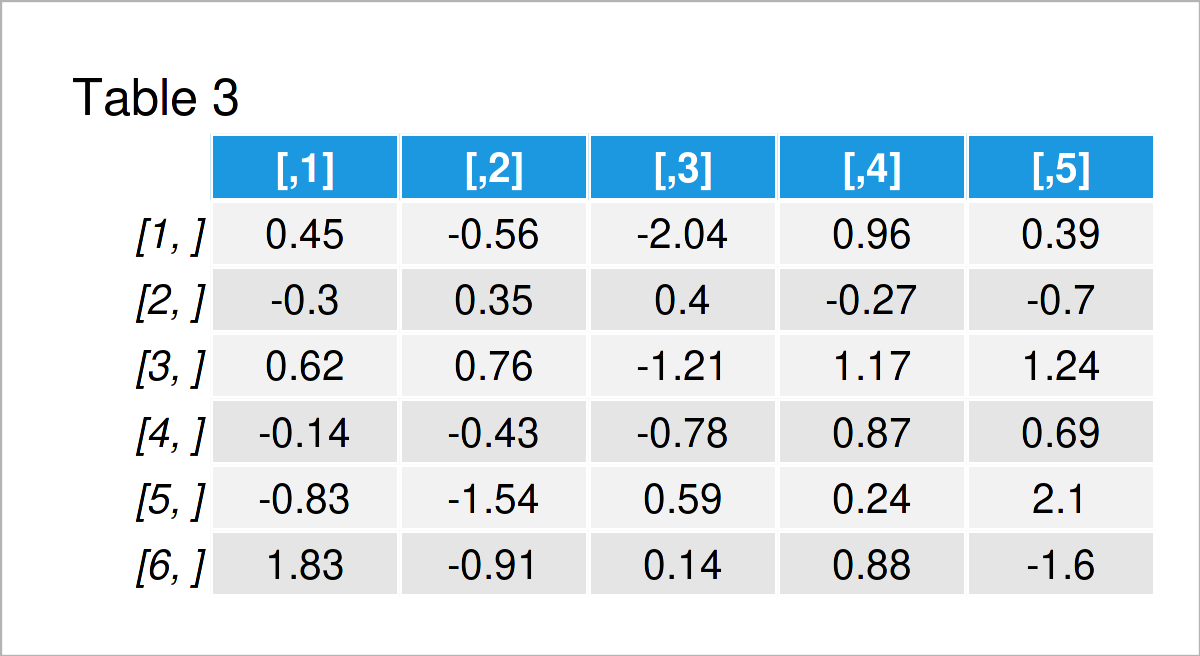# Round All Values in Matrix in R (2 Examples)

This tutorial explains how to round all values in a matrix object in R.

The tutorial will contain the following content blocks:

Let’s dive right in!

## Creation of Example Data

The first step is to create some exemplifying data:

```set.seed(354238654)                     # Set seed
mat <- matrix(rnorm(30), ncol = 5)      # Create random example matrix
mat                                     # Print random example matrix```Table 1 shows that our example data is constituted of six rows and five numeric columns. The values have not been rounded yet.

## Example 1: Round All Values in Matrix to Zero Digits

Example 1 illustrates how to round all matrix elements to zero digits using the default settings of the round function.

Consider the R programming code below:

```mat_round1 <- round(mat)                # Round to zero digits
mat_round1                              # Print rounded matrix```Table 2 shows the output of the previous code: Our matrix has been rounded to zero digits after the decimal point.

## Example 2: Round All Values in Matrix to N Digits

The following R programming syntax explains how to round the numeric values in a matrix to a certain number of digits.

For this task, we can use the digits argument of the round function as shown below:

```mat_round2 <- round(mat, digits = 2)    # Round to two digits
mat_round2                              # Print rounded matrix```The output of the previously shown R programming code is shown in Table 3 – A matrix where all values have been rounded to two digits.

## Video & Further Resources

Do you need further information on the R programming code of this tutorial? Then you could have a look at the following video tutorial on my YouTube channel. I explain the R programming syntax of this tutorial in the video.

Please accept YouTube cookies to play this video. By accepting you will be accessing content from YouTube, a service provided by an external third party.If you accept this notice, your choice will be saved and the page will refresh.

Furthermore, you may read the other tutorials on my website. I have published numerous articles already.

Summary: In this R tutorial you have learned how to round all elements in a matrix. In case you have further questions, let me know in the comments section below. Besides that, don’t forget to subscribe to my email newsletter in order to receive regular updates on new posts.

Subscribe to the Statistics Globe Newsletter